## Introduction

Quantum optomechanics has exhibited tremendous theoretical and experimental progress in recent years towards controlled manipulations of the interaction of cavity photons with mechanical oscillators1,2,3,4,5,6,7,8,9,10,11,12,13,14,15,16,17,18,19,20,21,22,23,24,25,26,27,28,29,30,31. A prominent application of such manipulations has been the cooling of the mechanical oscillator7,8,14 and the converse regime of the amplification of its motion16,20,21,22,23,24. Provided the total damping rate of the oscillator is negative, the laser-driven cavity mode can parametrically amplify the mechanical motion in a self-sustained limit-cycle of oscillations16,17,18,19,20,21,22,23,24,32 that has a nonclassical counterpart25,26,27,28,29,30.

Here we explore a different avenue, by raising the question: can the quantized oscillator transform thermal energy from a heat bath (rather than a laser) into mechanical work33,34,35,60 and thus act as a heat engine? Is such work similar to the self-induced oscillation discussed above? These questions pertain to a subtle and unsettled issue: what is the proper definition of work in a quantized (non-driven) heat engine and what limitations does thermodynamics set on its extraction?

We address these issues in the context of a realizable model that consists of a working medium, here the optical mode, constantly coupled to two distinct thermal baths and the mechanical oscillator that extracts the work. This is the standard situation in quantum open systems: our cavity mode constantly and unavoidably interacts with the outside electromagnetic vacuum (the cold bath) and with a spectrally filtered heat source (hot bath). This setup is an example of a continuous heat machine that35, contrary to more commonly known strokes-operated machines (such as the Carnot or Otto engine)4,34,35,36,37,38,39,40,41,42, does not involve decoupling from and recoupling to alternate (hot and cold) thermal baths. Continuous, fully quantized machines may therefore be operated completely autonomously, without external intervention, after launching them in a “push & go” fashion. While there is a well-established definition of work as long as the heat machine is driven by an external field (acting as a piston)35,36,43, it is more subtle to quantify work once this field is quantized. This subtlety is related to the fundamental question: How is the energy exchange between two quantum systems divided between heat and work?

Here we invoke the general and rigorous definition of maximal work storage (capacity) in the device: it is measured by the nonpassivity (see below)44,45,46 of the quantum state of the “piston”, here the mechanical oscillator. The “piston” interacts with the working medium (here the optical mode), which in turn is thermalized by the two baths. As the piston evolves, typically in a non-unitary fashion, its maximal work capacity (nonpassivity) changes. This rate of change is the maximal extractable power. In driven-piston scenarios34,35,36,37,38,39,40,41,42,43,47,48 work can be extracted by the piston from the system via unitary or classical operations. It is then independent of the initial state of the piston, which is not a thermodynamic resource. By contrast, we are concerned with work capacity in an autonomous, quantized setup that crucially depends on the initial state of the oscillator just as the work stored in an initially compressed spring. This initial state is then an extra thermodynamic resource quantified by nonpassivity, which is largely (but not completely) determined by its negentropy (see SI). This extra resource may be crudely viewed as an additional (pseudo) “bath” whose state-dependent effective temperature TM may be (for some time) arbitrarily low. Consequently, this extra resource may yield higher efficiency than the standard Carnot-cycle efficiency 1-Tc/Th, where the only resources are the hot and cold baths at temperatures Th and Tc, respectively. Consistency with the Second Law is ensured by construction (see SI). Not less important is the validity of this analysis for arbitrary nonunitary and nonadiabatic evolution43,49,50 in a quantized setup, since nonadiabatic (fast) evolution may yield much higher maximal power than standard stroke cycles that obey the quasiadiabatic Curzon-Ahlborn bound36,51. The present scenario has become timely, since the quantum state-preparation of the mechanical oscillator is now experimentally-feasible by optical pulses52,53.

## Model

We start from the basic optomechanical Hamiltonian wherein an optical cavity mode (denoted by O), is coupled to (cold and hot) two thermal baths and to a mechanical oscillator (denoted by M)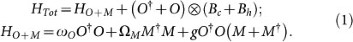Here O, O and M, M are the creation and annihilation operators of the cavity mode and the oscillator, respectively; ωO, ΩM and g are their respective frequencies and coupling rate. The cavity mode O directly interacts with two thermal baths: hot (Bh) and cold (Bc) baths with non-flat spectra, whereas the oscillator M is damped by a nearly-Markovian phonon bath. The response (coupling) spectra of the cold (c) and hot (h) baths may be broad, as opposed to a single-mode laser, but controllable, as detailed below: we shall take the hot bath to be a spectrally-filtered broadband source of thermal noise and the cold bath to be the vacuum that is coupled to the cavity mode, with typically a Lorentzian-shaped spectrum4,13 (Fig. 1b).

In particular, a toroid microcavity is a candidate for implementing the model mentioned above. While the microcavity losses are described by the coupling to the cold bath, a second hot bath could be coupled to the cavity through an optical fiber taper (see Fig. 1(a)).

## Analysis

We transform the operators to those of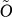,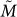, the mixed optical-mechanical modes that diagonalize HO+M without changing their frequency (see Methods). Then the interaction between the optical mode and the baths in (1) is found to indirectly affect the mechanical oscillator, enabling it to draw energy from the heat bath via the optical mode. Whereas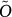rapidly reaches a steady state,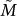keeps evolving thereby allowing its amplification. The evolution equation of their joint state has the form43,49,50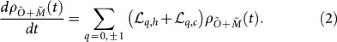Here q = 0, ±1 label the harmonics ωO, ω± = ωO ± ΩM, respectively and the Lindblad generators associated with these harmonics in the two baths,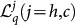, depend on the bath-response rates Gjq) (see Methods) In what follows we shall restrict ourselves to low excitations and linear amplification of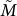and to the weak optomechanical coupling regime. Namely, we shall assume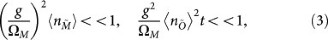where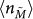and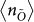are the mean numbers of quanta in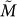and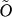, respectively. In this (quasi steady-state, linear-amplification) regime we can write a master equation for the slow dynamics of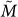(see Methods). Upon representing the reduced density matrix of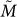,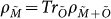in terms of coherent states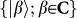,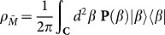, where P(β) is the quasi-probability distribution, this linearized master equation assumes the form of the Fokker-Planck equation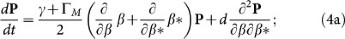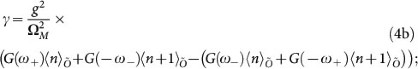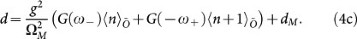Here G(ω) = Gc(ω) + Gh(ω) and ΓM and dM are the drift and diffusion rates produce by the direct interaction between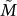and a phonon bath, while γ and d, are their counterparts due to the indirect coupling between M and the hot and cold bath, through O. They depend on the combined spectral response (coupling spectra) of the cold and hot baths, G(ω) = Gc(ω) + Gh(ω), sampled at the combination frequencies of O and M, ±ω± = ±(ω0 ± ΩM) and on the mean-number of quanta,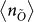at steady-state. While ΓM is always positive, γ may be also negative: γ is a sum of terms that involve the joint response of the two baths associated with the q = ±1 harmonics. Spectral separation of the two baths allows the negativity of the sum, as required for work extraction. When there is only one thermal bath with inverse temperature β and spectrum G(ω), γ is positive definite, i.e. no work is allowed.

### Energy amplification

The energy evolution of any initial state ofis found from (4) to be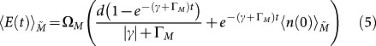where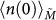is the mean initial number of oscillator quanta. Typically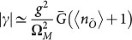where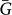is the averaged response bandwidth of the two baths coupled to the optical mode.

The condition γ + ΓM < 0, where ΓM is the oscillator damping rate by its environment (and not by the optical mode4,13), ensures the amplification of the oscillator energy,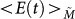. However, it does not represent work extraction: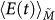is “blind” to the quantum state of the oscillator and does not discern work from heat (or noise) amplification. In what follows we monitor work extraction by the quantized oscillator and analyze its dependence on the evolving quantum state, based on nonpassivity.

### Work capacity and extraction as nonpassivity

For a given state of the oscillator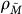, the work capacity is the maximum extractable work expressed by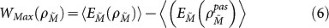Here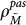is a passive state44,45, defined as a state that minimizes the mean energy of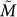, without changing its entropy and thus maximizes the work extractable from the state at hand,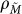. Equivalently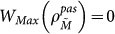, i.e., a passive state is a state in which work cannot be extracted. The passivity of a state is manifest by the monotonic decrease of its energy distribution P(E) from its value at the origin,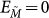44,45. Nonpassivity will be shown below to differ from known characteristics of quantum states, such as their purity or Wigner-function negativity54,55.

As the initial state of the oscillator,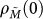, evolves (via a master equation56,59) to a state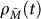, the maximum extractable work changes, according to Eq. (6), by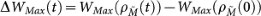. For an increase of the work capacity with time, it is necessary to prepare M in a nonpassive state and ensure that γ + ΓM < 0.

The upper bound for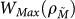is obtained by taking the lower bound of the second term in Eq. (6), i.e., setting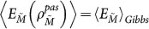, since the Gibbs state is the minimal-energy state with the same entropy as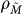33,34. This passive (effective “Gibbs”) state may be written as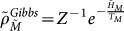. Its effective temperature TM is merely a parameter that characterizes the evolution of an arbitrary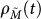.

Upon taking the time derivative of this upper bound of Eq. (6) and using the properties of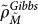, we find that the extractable power is maximized by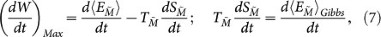where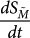is the entropy-production rate.

Equation (7) yields the efficiency bound upon dividing the output power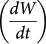by the heat-current input flowing from the hot bath43,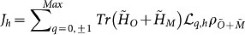where the sum is over the harmonics q,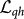are the corresponding Lindblad operators associated with Bh and ρO+M is the joint O + M density matrix. We then obtain the efficiency bound in the form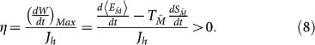The term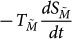on the r.h.s of (8), represents the effective heating rate which cannot be ignored for a quantum oscillator: it expresses the rate of loss of nonpassivity.

### Work extraction dependence on the quantum state

By contrast to energy extraction, work-capacity increaseWMax(t) > 0) requires an initially non-passive distribution in the (amplification) regime γ + ΓM < 0. We seek the conditions for maximal work extraction. A clue is provided upon introducing the low-temperature approximation to the entropy production rate in Eqs. (7),(8) for TM ≈ 0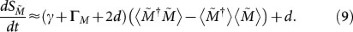The first term is strongly state-dependent, as shown in what follows.

1. 1

An initially coherent state, |β(0)〉, evolves in the linear amplification regime of the Fokker-Planck equation (4a) towards a distribution that is centered at an exponentially growing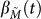and increasingly broadened by diffusion. The corresponding maximal work extraction is given by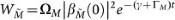. Thus, the coherent-state work capacity exponentially increases in this regime. The heating term (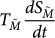) is minimized by this state at short times (according to (9)) and yields the optimal condition for work extraction. It is sustainable at long times, as an initial coherent state retains its nonpassivity and is never fully thermalized.For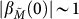the maximal efficiency bound η in Eq. (8) may exceed the standard Carnot bound, due to the slow rising entropy and effective temperature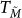. Eventually, the efficiency will drop below Carnot, since the effective temperature of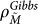rises due to diffusion, as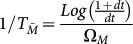. It is nevertheless significant that an initial small-amplitude coherent state allows to extract work over many cycles with an efficiency above the standard two-bath Carnot bound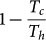. The extra efficiency has its origin in an extra thermodynamic resource (not present in the standard Carnot engine) that boosts the efficiency while complying with the second law of thermodynamics (see SI). In the quasiclassical limit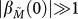the Carnot bound is recovered. Namely, the maximal power extraction determined by nonpassivity reproduces in the quasiclassical limit that of an externally (parametrically) modulated heat engine (proposed in Ref. 50) that obeys the standard cyclic work definition15.

2. 2

The evolution of an initial phase-averaged coherent state is obtained by integrating over the initial phase θ of a coherent state.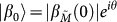, yielding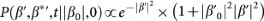where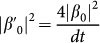. For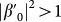this distribution is nonpassive, allowing exponentially growing work extraction.

3. 3

The Fock- state initial work capacity (ΩMnM(0)) does not increase with time, but rather decreases until the state becomes passive. The reason is the fast thermalization of a Fock state: its heating rate prevails over the rate of work production, so that the overall change in work capacity by an initial Fock state is always negative (Fig. 2).

Thus, as opposed to energy amplification (Eq. (5)), the extractable work crucially depends on the initial phase-plane distribution of the piston. Other nonpassive distributions, such as squeezed states or Schroedinger-cat states, can be shown to undergo faster entropy production and are therefore less optimal as far as work production is concerned.

### Comparison with self-induced oscillations

The regime of work extraction in an autonomous optomechanical heat engine (OHE) differs from the regime of self-induced oscillations (SIO) in its laser-powered counterpart in several salient respects:

1. 1

The OHE regime requires the coupling of the cavity mode to two distinct heat baths to ensure quasi-cyclic operation (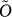evolves cyclically while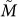does not), with clear thermodynamic bounds. The SIO-regime does not require such considerations.

2. 2

The SIO regime relies on a single-mode laser drive and does not occur under broadband driving. By contrast, OHE may be powered by broadband (albeit spectrally-filtered) hot and cold baths (Fig. 1).

3. 3

Perhaps most importantly, the SIO regime involves coherent (phase-locked) oscillator- and cavity- modes, whereas work extraction in the OHE may occur for a phase-averaged coherent-state of the oscillator, i.e., without any phase locking with another mode. Namely, work extraction, just as energy-extraction or SIO, requires γ + ΓM < 0. Yet, contrary to SIO, even in the classical limit the mechanical position need not evolve as x = x(0) + ACosΩMt, but can follow more general trajectories, such as those corresponding to a phase-averaged ensemble. On the other hand, the restriction of work extraction to nonpassive states has no counterpart in the SIO regime.

## Discussion

In the present paper we have proposed an autonomous (self-contained) optomechanical heat-engine, allowing for the hitherto unexplored role of the state of the quantized mechanical oscillator in the linear amplification regime, i. e., before the onset of saturation for large oscillator amplitudes.

These predictions may be tested for an optomechanical setup that may be powered by thermal noise filtered to eliminate its spectral overlap with the optical cavity mode. Desirable parameters are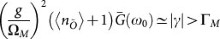. Taking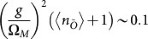, the requirement amounts to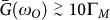. As an example, we take a mechanical oscillator with damping ΓM ~ 1 kHz, frequency ΩM ~ 3 MHz, optomechanical coupling g ~ 1 MHz,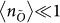(Boltzmann factor or mean thermal occupancy) of the optical cavity mode. The filtered heat bath is coupled to the cavity via band stop filter that can have an unlimited bandwidth but a sharp lower cut off of few MHz width Fig. 1(b). The EM vacuum has a cavity bandwidth ~1 GHz. Work-extraction rate has been related to the nonpassivity of the oscillator state, the only rigorously justifiable measure of work extraction in time-independent autonomous setups44,45. It is shown here to crucially depend on the initial quantum state, in contrast to mean-energy amplification. The resulting efficiency bound (8) involves the effective temperature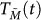. As long as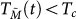, Eq. (8) may surpass the standard two-bath Carnot bound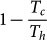. Because it complies with Spohn's inequality57 (see SI), the present (evolving) efficiency bound is consistent with the second law. It shows that the piston may serve as a low-entropy resource which is excluded by the standard (classical-parametric) limit of work extraction. It comes about upon allowing for the inevitable but commonly ignored entropy growth of the quantum oscillator and its linear amplification at finite times.

The efficiency and work-production rate (power) derived by us are both practically and conceptually interesting, since the initial “charging” of the oscillator by quantum state-preparation is sought to be maximally efficient for subsequent operation. Such preparation is a one-time investment of energy and does not invalidate the work and its extra efficiency obtained in the linear-amplification regime. In this respect, our analysis has yielded nontrivial results: (a) As the initial coherent amplitude of the oscillator decreases, the resulting efficiency increases, although the entropy growth of the oscillator might then be expected to reduce (rather than enhance) the efficiency. (b) Work extraction obtained from an initial coherent-state has been found to be superior to that of other states, because of its larger sustainable nonpassivity, conditioned on its low heating or entropy-production rate: This is consistent with the coherent state being the “pointer-state” of the evolution58. (c) Not less remarkable is that, in contrast to laser-powered self-induced oscillations, broadband (heat-) powered work extraction does not require coherence or phase-locking: an initial phase-averaged coherent state still yields work extraction.

## Methods

### The dressing information

The dressing transformation can be expressed in terms of new variables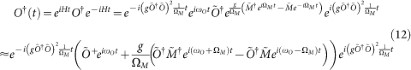The operator O + O which appears in the interaction Hamiltonian is given in terms of new dynamical variables as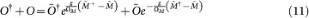The Heisenberg-picture Fourier decomposition of O + O within the lowest order approximation with respect to a small parameter gM, can be obtained from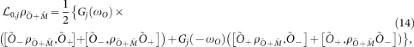The approximation made in (12) is valid under Eq. (3).

We then have in the interaction picture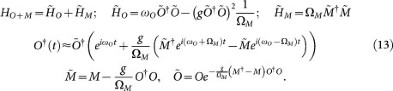### The Master equation

The master equation (3) has the form43,49,50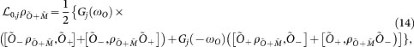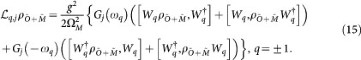Here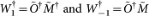The generator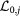drives the evolution of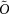, which is faster than that of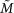whose evolution is governed by the Lindblad generators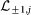.

### The partially stationary regime

The evolution governed by (14) and (15) has two timescales. The slow one, that includes all the terms multiplied by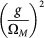is related to changes in the state of M, while the fast one governs changes in the system.

The fast evolution equation for the diagonal elements of the system is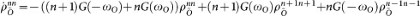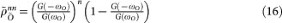with average population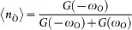, where G(ω) = Gh(ω) + Gc(ω), being the bath response spectra. Under these conditions, the master equation for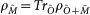may be rewritten in the Fokker-Planck form (4).